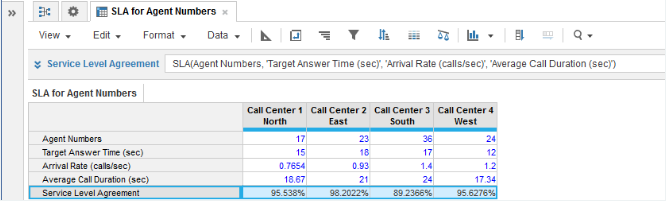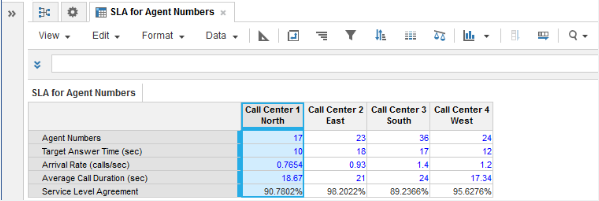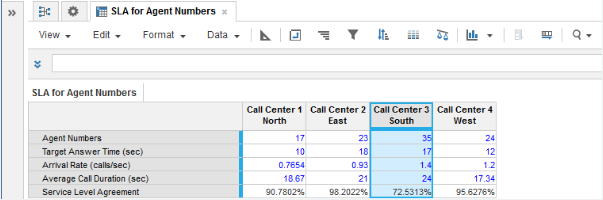1. Calculation functions
2. All Functions
3. Call Center Planning Functions
4. SLACalculates the percentage of incoming calls to be answered within a target answer time to meet a Service Level Agreement.

Equation:

SLA is the solution to this equation:

1- ERLANGC(x,a)e(a-x)t/z, where SLA ≠ 1

where:

• a = offered load (call arrival rate multiplied by average call duration = y*z)

## Syntax

SLA(x, t, y, z)

where:

• x: Number of servers, telephone lines, or agents
• y: arrival rate of calls or the number of calls per unit of time
• z: the average call duration

## Format

Input Format Output Format

x: Number (no time formatting)

t: Number (no time formatting)

y: Number (no time formatting)

z: Number (percentage)

Number (percentage)

## Arguments

The function uses the following arguments:

• x: Number: Numeric line item, property, or expression
• t: Number: Numeric line item, property, or expression
• y: Number: Numeric line item, property, or expression
• z: Number: Numeric line item, property, or expression

## Constraints

The function has the following constraints:

• All parameters are mandatory.
• All parameters must be a numeric line item, property, or expression.
• Time unit used for t, y, and z must be the same. For example, all units are set in seconds—the cells are not time-formatted.
• Result line item must be numeric (percentage).
• Maximum number of agents is five million.

## Excel equivalent

• No Excel equivalent

## Example

We have four call centers each covering a separate geographical area:

• Each call center must achieve a target answer time. Target Answer Time (sec) is a numeric source line item for entering the target answer time for incoming calls.
• The arrival rate of incoming calls and the average call duration are known for each call center from historical monitoring of operational performance. These metrics are entered in numeric source line items for Arrival Rate (calls/sec) and Average Call Duration.
• Agent Numbers is a source line item for entering the agent staffing level at each call center.

We can use the SLA function in the Service Level Agreement numeric result line item to calculate the percentage of incoming calls that will be answered in the target answer time:In this example, we use the SLA function to perform what-if analysis for the target answer times you plan to maintain at call centers. Here the target answer time for Call Center 1 North has been decreased from 15 to 10 seconds, resulting in an approximate 5% reduction in the service level agreement level that can be maintained with the same number of agents:Alternatively, you might want to adjust the number of agents available at a call center while keeping to the original target answer time. Here the number of agents at Call Center 3 South has been reduced from 36 to 35 with a consequent reduction of approximately 17% in the service level agreement level that can be maintained for the same target answer time: